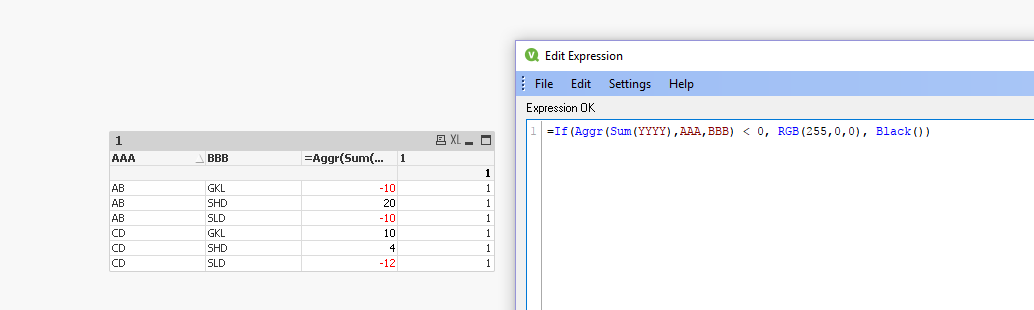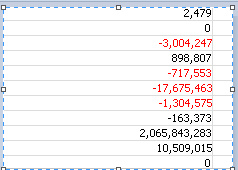# QlikView App Dev

Discussion Board for collaboration related to QlikView App Development.

Announcements
Skip the ticket, Chat with Qlik Support instead for instant assistance.
cancel
Showing results for
Did you mean:Creator III

## Text color in red for calculated dimension

Hi,

I have a calculated dimension like the following

aggr(sum({<xxx=,Date={"\$(vVariable)"}>}YYYY),AAA,BBB))

I want to print negative value in Red. so in text color I put the below code

if(  aggr(sum({<xxx=,Date={"\$(vVariable)"}>}YYYY),AAA,BBB)))<0,RGB(255,0,0),black()

As a result I am getting some negative value as red and some negative value are not in red. This is very strange .

Even if I put NUM function before the aggr the result is same.

Any help much appreciated.

28 RepliesMVP

I may not be having all possible scenarios, but I tried with a very simple sample and I don't see any issues, expect some misplaced parenthesis which could be typos as you mentioned that it worked in some of the casesWould you be able to share a sample where you can show that it isn't working?Master II

Hi Ashis,

In that case create this dimension in script: The issue is happening because at dimension level the aggregation is not happening as expected.

Script:

Key, //create a key to join with the main table

AAA as AAA1 //to eliminate synthetic key

BBB as BBB1,

sum(YYYY) as Dimension 1

resident table

group by key,AAA, BBB;

No in the pivot use Dimension 1 filed as dimension.

Br,

KC

Best Regards,
KCCreator III
Author

Hi Sunny,

In my pivot chart I have few calculated dimensions and few expressions . I think problem is it is not properly interpreting the numeric value.

for some it is showing correct and for sum it is not showing correct.

dimension =num(aggr(sum({<xxx=,Date={"\$(vVariable)"}>}YYYY),AAA,BBB,CCC)),'#,##0;-#,##0')

color code :

if(sum(aggr(sum({<xxx=,Date={"\$(vVariable)"}>}YYYY),AAA,BBB,CCC)))<0RGB(255,0,0),black())

I tried with few other functions such as

if(subfield(trim(text(sum(aggr(sum({<xxx=,Date={"\$(vVariable)"}>}YYYY),AAA,BBB,CCC))))),1)='-1',rgb(255,0,0),black())

However it is not working.MVP

Won't really know unless I see a sample...Master II

dimension= aggr(sum({<xxx=,Date={"\$(vVariable)"}>}YYYY),AAA,BBB)

color

=if(  aggr(sum({<xxx=,Date={"\$(vVariable)"}>}YYYY),AAA,BBB)=fabs(aggr(sum({<xxx=,Date={"\$(vVariable)"}>}YYYY),AAA,BBB)),black(),RGB(255,0,0)    )

regards

Learning never stops.Creator III
Author

It was great idea, however for some value it is not working

See the attached image.Anonymous
Not applicable

try this,

if(  left(aggr(sum({<xxx=,Date={"\$(vVariable)"}>}YYYY),AAA,BBB),1)='-', red(),black())Creator III
Author

Hi John,

I had tried that one too, it does not work.Creator III
Author

It worked now.

Aggr function does not work in calculated dimension how I was using, so removing aggr , gave me correct result.Community Browser